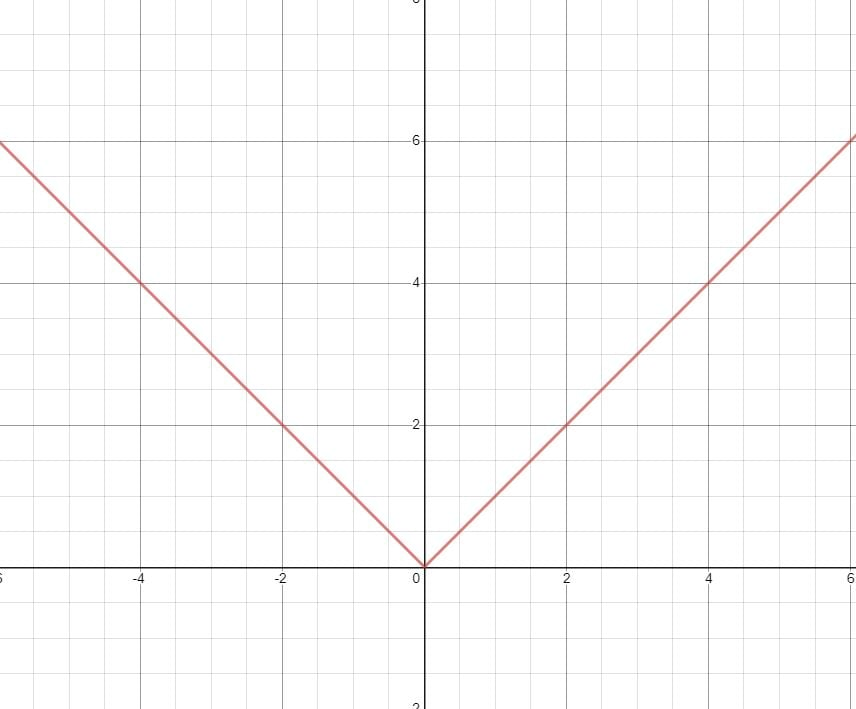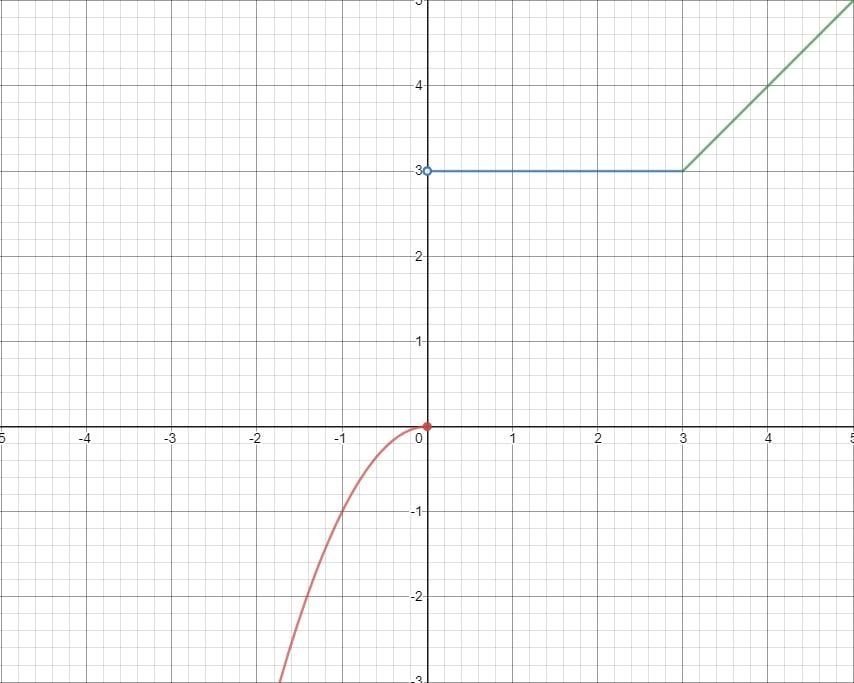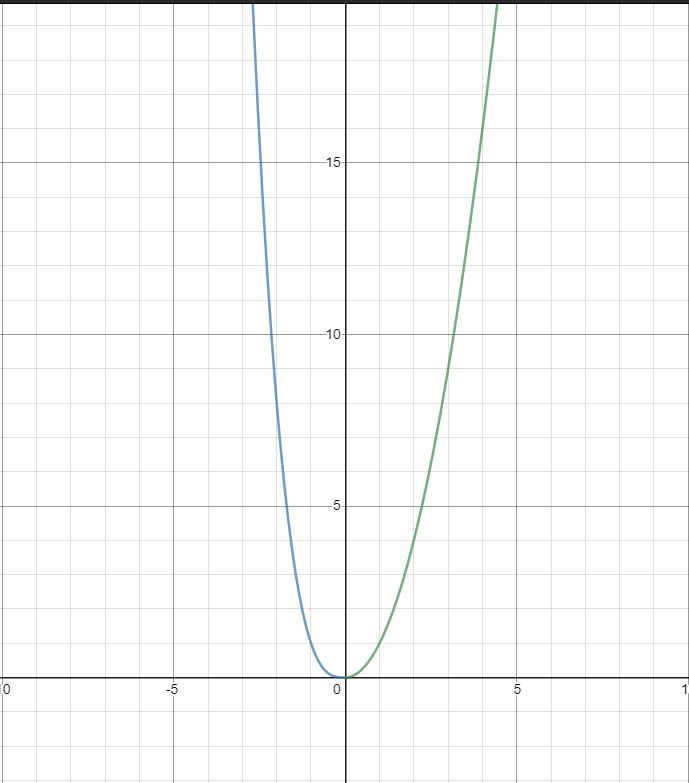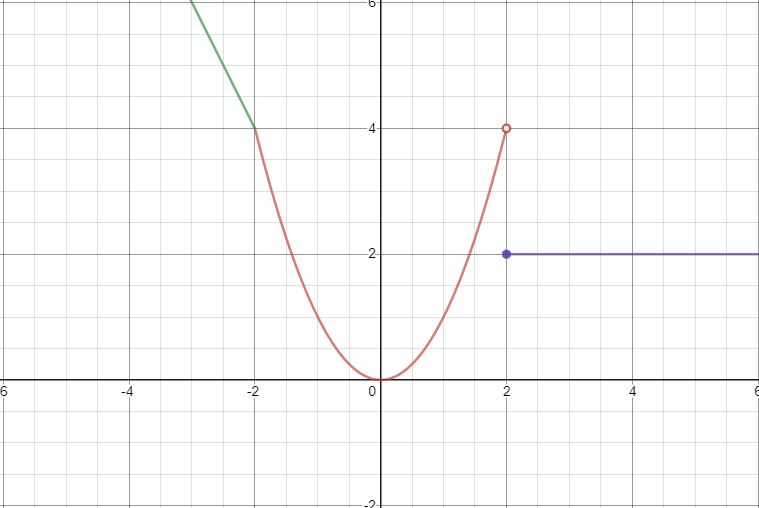# Part 4: Piecewise Functions | Year 11 Further Functions and Relations

Piecewise functions are very useful in the real world. However, it can be quite confusing to understand! So, in this article, we will explain everything you need to know to ace piecewise functions!Do you feel that your understanding Piecewise Functions looks like a Piecewise Function? Don’t worry! In this article, we’re going to fill in the blanks of your understanding of Piecewise Functions.

## Year 11 Advanced Mathematics: Piecewise Functions

This topic allows us to understand functions which have different definitions for different input values.

This is a very useful idea as many real-world systems are not defined the same for all inputs, unlike most polynomials we have seen so far.

An example to illustrate this is tax. the formula for calculating tax is different depending on a person’s income rather than being the same for everyone.

## NESA Syllabus Outcomes

NESA expects students to demonstrate proficiency in the following syllabus outcomes;

• Model, analyse and solve problems involving quadratic functions
• Solve practical problems involving a pair of simultaneous linear and/or quadratic functions algebraically and graphically, with or without the aid of technology; including determining and interpreting the break-even point of a simple business problem

## Assumed Knowledge

Students should already be familiar with function notation, domain and range, inequalities and evaluating and sketching polynomials.

## Definition and Evaluating Piecewise Functions

A piecewise function is a function defined separately for different intervals of $$x$$ – values (different domains).

The simplest example to illustrate this is the absolute value function, defined by:

$$y = f(x)= \begin{cases} -x & ;x<0 \\ x & ;x \geq 2 \end{cases}$$

This means that:

1. $$f(x) = -x$$ when $$x$$ is less than $$0$$
2. $$f(x) = x$$ when $$x$$ is greater than or equal to $$0$$

Graphically this is:Evaluating a piecewise function only has one extra step when compared to a regular function, which is to determine which region we are in.

Simply determine which of the inequalities our $$x$$ value is consistent with, and you have the equation of the function! Evaluating is now trivial, as all we need to do is substitute in our $$x$$ value.

Considering our absolute value example:
For $$x = 5$$ we are in the region $$x \geq 0$$, so the function is $$f(x) = x$$ and $$y = f(5) = 5$$
For $$x = -5$$ we are I the region $$x < 0$$, so the function is $$f(x) = -x$$ and $$y = f(-5) = 5$$

Always be careful when considering values at the edge of a region! Make sure that you choose the correct region based on whichever domain includes the $$x$$ value (i.e. $$\geq$$ rather than $$>$$).

This is illustrated by the discontinuity in the next section.

## Sketching Piecewise Functions

Sketching piecewise functions can similarly be made quite easy by considering it as sketching multiple separate functions.

As an example, we will go through the process of sketching:

$$y = f(x)= \begin{cases} -x^2 & ;x \leq 0 \\ 3 & ;0<x<3 \\ x & ;x \geq 3 \end{cases}$$

This may look complicated, but like our first example we can break this down to:

$$y = – x^2$$ when $$x \leq 0$$
$$y = 3$$ when $$x$$ is between $$0$$ and $$3$$
$$y = x$$ when $$x \geq 3$$

And now we have $$3$$ functions defined over $$3$$ separate domains. These are all simple functions and should be recognised as a parabola and $$2$$ straight lines, which we already know how to sketch.

Sketch each function in their respective domains, and you have sketched the piecewise function!An important feature to note is the discontinuity, where the different parts of the function do not meet each other, as at $$x = 0$$ in our example.

It is important to indicate which point is a part of the function, and which is not, as we cannot have multiple $$y$$ values for the same $$x$$ value (a piecewise function is still a function).

This is denoted by using a closed or open circle as you would have done when graphing inequalities on a number line.

## Want to avoid your Year 11 Maths Advanced progress looking like a Piecewise Function?

Matrix+ online Year 11 Maths Avdanced courses are the expert guided solution to your Maths problems. Learn more.## Concept Check Questions

1. Evaluate the following:

$$y = f(x)= \begin{cases} x^5+3x^2+5 & ;x \leq 3 \\ 6-3x^3 & ;x >3 \end{cases}$$

2. Sketch the following:

$$f(x)= \begin{cases} -x^3 & ;x>0 \\ x^2 & ;x \leq 0 \end{cases}$$

3. Sketch the following:

$$f(x)= \begin{cases} 2 & ;x \geq 2 \\ x^2 & ;-2<x<2 \\ -2x & ;x \leq -2 \end{cases}$$

## Solutions

1. $$f(0) = 5, f(5) = -369, f(3) = 275$$

2.3.## Want to check your progress with Piecewise Functions?© Matrix Education and www.matrix.edu.au, 2021. Unauthorised use and/or duplication of this material without express and written permission from this site’s author and/or owner is strictly prohibited. Excerpts and links may be used, provided that full and clear credit is given to Matrix Education and www.matrix.edu.au with appropriate and specific direction to the original content.